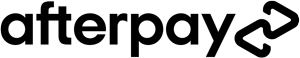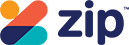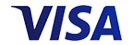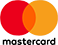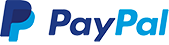Grid List\$59.95
Increase value Decrease value
WAS \$29.95 NOW \$14.95
Increase value Decrease value\$49.95
Increase value Decrease value\$47.95
Increase value Decrease value\$49.95
Increase value Decrease value\$39.95
Increase value Decrease value\$39.95
Increase value Decrease value
WAS \$19.95 NOW \$9.98
Increase value Decrease value
WAS \$16.95 NOW \$12.95
Increase value Decrease value\$47.95
Increase value Decrease value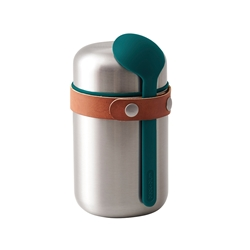\$59.95
Increase value Decrease value
WAS \$19.95 NOW \$9.98
Increase value Decrease value